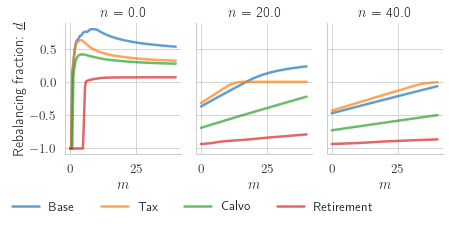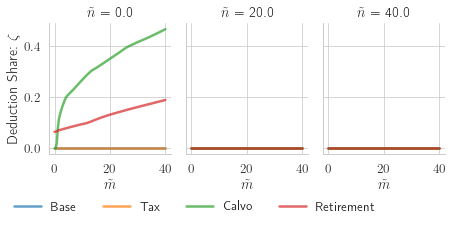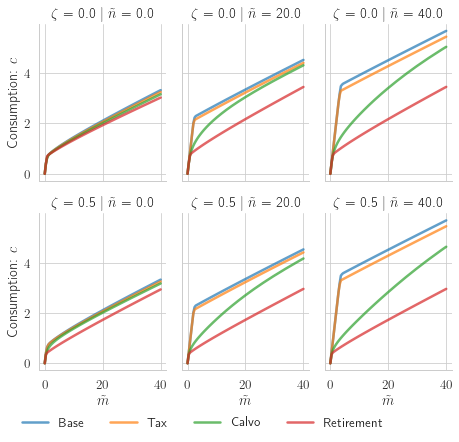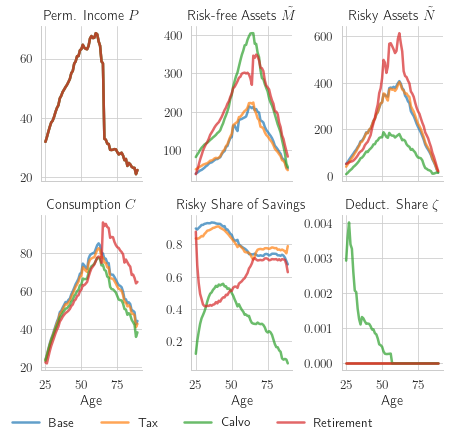# A Two-Asset Savings Model with an Income-Contribution Scheme

## Johns Hopkins University

This notebook demonstrates the use of the RiskyContrib agent type of the HARK Toolkit. The model represents an agent who can save using two different assets---one risky and the other risk-free---to insure against fluctuations in his income, but faces frictions to transferring funds between assets. The flexibility of its implementation and its inclusion in the HARK toolkit will allow users to adapt the model to realistic life-cycle calibrations, and also to embedded it in heterogeneous-agents macroeconomic models.

In :
# Preamble

from HARK.ConsumptionSaving.ConsRiskyContribModel import (
RiskyContribConsumerType,
init_risky_contrib_lifecycle,
)
from time import time
from copy import copy

import numpy as np
import seaborn as sns
import pandas as pd
from Simulations.tools import pol_funcs_dframe, age_profiles
import os

# This is a jupytext paired notebook that autogenerates a .py file
# which can be executed from a terminal command line
# But a terminal does not permit inline figures, so we need to test jupyter vs terminal
# Google "how can I check if code is executed in the ipython notebook"
from IPython import get_ipython # In case it was run from python instead of ipython

# If the ipython process contains 'terminal' assume not in a notebook
def in_ipynb():
try:
if 'terminal' in str(type(get_ipython())):
return False
else:
return True
except NameError:
return False

# Determine whether to make the figures inline (for spyder or jupyter)
# vs whatever is the automatic setting that will apply if run from the terminal
if in_ipynb():
# %matplotlib inline generates a syntax error when run from the shell
# so do this instead
get_ipython().run_line_magic('matplotlib', 'inline')
else:
get_ipython().run_line_magic('matplotlib', 'auto')


## Model Description¶

I now discuss the main components of the model informally, and leave its full recursive mathematical representation for Section \ref{sec:recursive}.

### Time, mortality, and utility¶

Time advances in discrete steps that I will index with $t$. The model can be used in both infinite and finite-horizon versions.

Agents face an exogenous risk of death $\delta_t$ each period, which becomes certain at the maximum age of the finite-horizon version. There are no intentional bequests; agents will consume all of their resources if they reach the last period, but they can leave accidental bequests upon premature death.

In each period, agents derive utility from consumption only. Their utility function follows a constant relative risk aversion specification. Formally, for a level of consumption $C$, the agent derives instant utility \begin{equation} u(C) = \frac{C^{1-\rho}}{1- \rho}. \end{equation}

#### Income process¶

Agents supply labor inelastically. Their labor earnings $Y_{i,t}$ are the product of a permanent component $P_{i,t}$ and a transitory stochastic component $\theta_{i,t}$ as in \cite{Carroll1997qje}, where $i$ indexes different agents. Formally, \begin{equation*} \begin{split} \ln Y_{i,t} &= \ln P_{i,t} + \ln \theta_{i,t} \\ \ln P_{i,t} &= \ln P_{i,t-1} + \ln \Gamma_{i,t} + \ln \psi_{i,t} \end{split} \end{equation*} where $\Gamma_{i,t}$ is a deterministic growth factor that can capture life-cycle patterns in earnings, and $\ln \psi_{i,t}\sim \mathcal{N}(-\sigma^2_{\psi,t}/2, \sigma_{\psi,t})$ is a multiplicative shock to permanent income\footnote{The mean of the shock is set so that $E[\psi_{i,t}] = 1$.}.

The transitory component $\theta_{i,t}$ is a mixture that models unemployment and other temporal fluctuations in income as \begin{equation*} \ln\theta_{i,t} = \begin{cases} \ln \mathcal{U}, & \text{With probability } \mho\\ \ln \tilde{\theta}_{i,t}\sim\mathcal{N}(-\sigma^2_{\theta,t}/2, \sigma_{\theta,t}), & \text{With probability } 1-\mho, \end{cases} \end{equation*} with $\mho$ representing the probability of unemployment and $\mathcal{U}$ the replacement factor of unemployment benefits.

This specification of the income process is parsimonious and flexible enough to accommodate life-cycle patterns in income growth and volatility, transitory unemployment and exogenous retirement. Introduced by \cite{Carroll1997qje}, this income specification is common in studies of life-cycle wealth accumulation and portfolio choice; see e.g., \cite{Cagetti2003jbes,Cocco2005rfs,Fagereng2017jof}. The specification has also been used in studies of income volatility such as \cite{Carroll1992bpea,Carroll1997jme,Sabelhaus2010jme}, which have yielded calibrations of its stochastic shocks' distributions.

#### Financial assets and frictions¶

Agents smooth their consumption by saving and have two assets available for this purpose. The first is a risk-free liquid account with constant per-period return factor $R$. The second has a stochastic return factor $\tilde{R}$ that is log-normally distributed and independent across time. Various interpretations such as stocks, a retirement fund, or entrepreneurial capital could be given to the risky asset. Importantly, consumption must be paid for using funds from the risk-free account. The levels of risk-free and risky assets owned by the agent will both be state variables, denoted with $M_{i,t}$ and $N_{i,t}$ respectively.

Portfolio rebalancing takes place by moving funds between the risk-free and risky accounts. These flows are one of the agents' control variables and are denoted as $D_{i,t}$, with $D_{i,t}>0$ representing a movement of funds from the risk-free to the risky account. Withdrawals from the risky account are subject to a constant-rate tax $\tau$ which can represent, for instance, capital-gains realization taxes or early retirement-fund withdrawal penalties. In sum, denoting post-rebalancing asset levels with $\tilde{\cdot}$, \begin{equation*} \begin{split} \tilde{M}_{i,t} &= M_{i,t} - D_{i,t}(1 - 1_{[D_{i,t}\leq0]}\tau)\\ \tilde{N}_{i,t} &= N_{i,t} + D_{i,t}. \end{split} \end{equation*}

At any given period, an agent might not be able to rebalance his portfolio. This ability is governed by an exogenous stochastic shock that is realized at the start of the period

\begin{equation*} \Adj_t \sim \text{Bernoulli}(p_t), \end{equation*}

with $\Adj_t=1$ meaning that the agent can rebalance and $\NAdj_t=1$ ($\Adj_t = 0$) forcing him to set $D_{i,t} = 0$. This friction is a parsimonious way to capture the fact that portfolio rebalancing is costly and households do it sporadically. Recent studies have advocated for \cite{Giglio2021aer} and used \cite{Luetticke2021aej_macro} this kind of rebalancing friction.

To partially evade the possibility of being unable to rebalance their accounts, agents can use an income deduction scheme. By default, labor income ($Y_{i,t}$) is deposited to the risk-free liquid account at the start of every period. However, agents can pre-commit to have a fraction $\Contr_t\in[0,1]$ of their income diverted to their risky account instead. This fraction can be tweaked by the agent whenever $\Adj_t = 1$; otherwise it stays at its previous value, $\Contr_{t+1} = \Contr_t$.

#### Timing¶The previous figure summarizes the timing of stochastic shocks and optimizing decisions that occur within a period of the life cycle model.

### Recursive representation of the model¶

Individual subscripts $i$ are dropped for simplicity. The value function for an agent who is not allowed to rebalance his portfolio at time $t$ is

\begin{equation*} \begin{split} V^{\NAdj}_{t}(M_t, N_t, P_t, \Contr_t) = \max_{C_t} u(C_t) + p_{t+1} &\beta\delta_{t+1} E_t \left[ V^{\Adj}_{t+1}\left( M_{t+1}, N_{t+1}, P_{t+1} \right)\right] +\\ \left(1-p_{t+1}\right) &\beta\delta_{t+1} E_t\left[V^{\NAdj}_{t+1}\left(M_{t+1}, N_{t+1}, P_{t+1}, \Contr_{t+1}\right) \right]\\ \text{Subject to:} \quad &\\ 0\leq& C_t \leq M_t \\ A_t =& M_t - C_t \\ M_{t+1} =& R A_t + (1-\Contr_{t+1}) Y_{t+1}\\ N_{t+1} =& \tilde{R}_{t+1}N_t + \Contr_{t+1}Y_{t+1}\\ P_{t+1} =& \Gamma_{t+1} \psi_{t+1} P_{t}\\ Y_{t+1} =& \theta_{t+1} P_{t+1}\\ \Contr_{t+1} =& \Contr_t \end{split} \end{equation*}

and that of agent who is allowed to rebalance is

\begin{equation*} \begin{split} V^{\Adj}_{t}(M_t, N_t, P_t) = \max_{C_t,D_t,\Contr_{t+1}} u(C_t) + p_{t+1} &\beta\delta_{t+1} E_t \left[ V^{\Adj}_{t+1}\left( M_{t+1}, N_{t+1}, P_{t+1} \right)\right] +\\ \left(1-p_{t+1}\right) &\beta\delta_{t+1} E_t\left[V^{\NAdj}_{t+1}\left(M_{t+1}, N_{t+1}, P_{t+1}, \Contr_{t+1}\right) \right]\\ \text{Subject to:} \quad &\\ \quad -N_t \leq D_t \leq M_t, \quad \Contr_{t+1} \in& [0,1], \quad 0 \leq C_t \leq \tilde{M}_t\\ \hfill\\ \tilde{M}_t =& M_t - D_t\left(1-1_{[D_t\leq0]}\tau\right)\\ \tilde{N}_t =& N_t + D_t\\ A_t =& \tilde{M}_t - C_t \\ M_{t+1} =& R A_t + (1-\Contr_{t+1}) Y_{t+1}\\ N_{t+1} =& \tilde{R}_{t+1} \tilde{N}_t + \Contr_{t+1}Y_{t+1}\\ P_{t+1} =& \Gamma_{t+1}\psi_{t+1} P_{t}\\ Y_{t+1} =& \theta_{t+1} P_{t+1} \end{split} \end{equation*}

## Parametrizations¶

This notebook will only demonstrate a life-cycle calibration of the model. However, the current implementation of the model is able to find and use the solution to infinite-horizon formulations (see the document in this repository for details).

For the present exercise, I calibrate the model's mortality and income paths to represent individuals who enter the model at age 25, retire exogenously at 65, and live to a maximum age of 90. Survival probabilities ($\delta$) come from the 2004 SSA life-tables for males. Income growth factors and volatilities ($\Gamma$, $\sigma_\psi$, $\sigma_\theta$) come from the calibration for high-school graduates in \cite{Cagetti2003jbes}.

To highlight the effect of different financial frictions on wealth accumulation and portfolio choice, I consider different configurations for the risky-withdrawal tax $\tau$ and the probability of being able to rebalance $p$:

\begin{itemize} \item \textbf{Base}: the probability of being able to rebalance is $p = 1$ and the risky withdrawal tax rate is $\tau = 0$, both constant throughout the agents' lives. \item \textbf{Tax}: the risky withdrawal tax is constant at $10\%$ and the agents can always rebalance their portfolios. \item \textbf{Calvo}: there is no risky withdrawal tax, but there is only a $25\%$ chance that agents can rebalance their portfolios every year. \item \textbf{Retirement}: there is no risky withdrawal tax, but the agents' ability to rebalance their portfolio is time-varying; they can rebalance their assets and pick their income-deduction scheme for certain when they enter the model at age 25, but then they have no chance of doing so again ($p=0$) until they retire. After retirement at age 65, they can always rebalance their portfolio ($p=1$). \end{itemize}

The rest of the parameters take the following values

Name in HARK Mathematical Symbol Value
CRRA $\rho$ $5.0$
Rfree $R$ $1.03$
DiscFac $\beta$ $0.9$
UnempPrb $\mho$ $0.05$
IncUnemp $\mathcal{U}$ $0.3$
RiskyAvg $E[\tilde{R}]$ $1.08$
RiskyStd $\sqrt{V[\tilde{R}]}$ $0.18$
In :
# Base calibration setup

# Make the problem life-cycle
par_LC_base = init_risky_contrib_lifecycle.copy()

# Turn off aggregate growth
par_LC_base['PermGroFacAgg'] = 1.0

# and frictionless to start
par_LC_base["tau"] = 0.0

# Make contribution shares a continuous choice
par_LC_base["DiscreteShareBool"] = False
par_LC_base["vFuncBool"] = False

# Make grids go up to higher levels of resources
# (one of the calibration produces high levels of nNrm)
par_LC_base.update({"mNrmMax": 500, "nNrmMax":1000})

In :
# Alternative calibrations

# Tax
par_LC_tax = copy(par_LC_base)
par_LC_tax["tau"] = 0.1

# Calvo
par_LC_calvo = copy(par_LC_base)

# Retirement
par_LC_retirement = copy(par_LC_base)
par_LC_retirement["AdjustPrb"] = [1.0] + [0.0]*39 + [1.0]*25
par_LC_retirement["tau"] = [0.0]*41 + [0.0]*24
par_LC_retirement["UnempPrb"] = 0.0


## Solution¶

With the parametrizations created, I now create and solve the agents.

In :
agents = {
"Base": RiskyContribConsumerType(**par_LC_base),
"Tax": RiskyContribConsumerType(**par_LC_tax),
"Calvo": RiskyContribConsumerType(**par_LC_calvo),
"Retirement": RiskyContribConsumerType(**par_LC_retirement),
}

for agent in agents:

print("Now solving " + agent)
t0 = time()
agents[agent].solve(verbose=True)
t1 = time()
print("Solving " + agent + " took " + str(t1 - t0) + " seconds.")

Now solving Base
Finished cycle #1 of 1 in 37.66172480583191 seconds.
Solving Base took 37.66172480583191 seconds.
Now solving Tax
Finished cycle #1 of 1 in 36.05218529701233 seconds.
Solving Tax took 36.05218529701233 seconds.
Now solving Calvo
Finished cycle #1 of 1 in 84.95266103744507 seconds.
Solving Calvo took 84.95266103744507 seconds.
Now solving Retirement
Finished cycle #1 of 1 in 53.0900604724884 seconds.
Solving Retirement took 53.0900604724884 seconds.


## Policy function inspection¶

Once agents have been solved, we can use their policy functions $\dFrac_t(m,n)$, $\Contr_t(\tilde{m},\tilde{n})$, and $c_t(\tilde{m},\tilde{n}, \Contr)$ for any period $t$.

In :
# Example
calib = "Base"
t = 0
print(agents[calib].solution[t].stage_sols["Cns"].cFunc(1.2,0.8,0.5))

0.1280581440929615
0.0
0.7103217030091933


I now illustrate the policy functions of different calibrations for an arbitrary period graphically.

Note that the solution algorithm represents the three simultaneous decisions that an agent can take as happening sequentially in ''stages'', in the order Rebalancing -> Income deduction share -> Consumption. See the document in this repository for more details.

In :
# Setup
from HARK.utilities import (
determine_platform,
test_latex_installation,
setup_latex_env_notebook,
)

pf = determine_platform()
try:
latexExists = test_latex_installation(pf)
except ImportError:  # windows and MacOS requires manual install
latexExists = False

setup_latex_env_notebook(pf, latexExists)

# General aesthetics
sns.set_style("whitegrid")
sns.set_context("paper", font_scale=1.5, rc={"lines.linewidth": 2.5})

# Parameters to feed to policy functions
t = 10
age = t + 24
mNrmGrid = np.linspace(0, 40, 100)
nNrm_vals = np.array([0.0, 20.0, 40])
Share_vals = np.array([0.0, 0.5])

# Evaluate policy functions
polfuncs = pol_funcs_dframe(agents, t, mNrmGrid, nNrm_vals, Share_vals)


### Rebalancing¶

The solution to this stage problem will be the policy function $d_t(\cdot, \cdot)$ that gives the optimal flow from risk-free to risky assets, which can be negative. However, it is convenient to define a normalized policy function $\dFrac_t$ as \begin{equation*} \dFrac_t(m, n) = \begin{cases} d_t(m,n)/m, & \text{ if } d_t(m,n) \geq 0 \\ d_t(m,n)/n, & \text{ if } d_t(m,n) < 0 \end{cases} \end{equation*} so that $-1 \leq \dFrac(m,n) \leq 1$ for all $(m,n)$.

In :
# Rebalancing fraction
polfuncs["$n$"] = polfuncs["n"]
g = sns.FacetGrid(
polfuncs[polfuncs.control == "dfrac"],
col="$n$",
hue="model",
height=3,
aspect=(7 / 3) * 1 / 3,
)
g.map(sns.lineplot, "m", "value", alpha=0.7)
g.add_legend(bbox_to_anchor=[0.5, 0.0], ncol=4, title="")
g.set_axis_labels("$m$", "Rebalancing fraction: $\dFrac$")

Out:
<seaborn.axisgrid.FacetGrid at 0x1c62cd48220>### Income deduction share¶

In :
# After rebalancing, m and n turn to their "tilde" versions. Create ntilde
# just for seaborn's grid labels.
polfuncs["$\\tilde{n}$"] = polfuncs["n"]
polfuncs["$\\Contr$"] = polfuncs["Share"]

# Share fraction
g = sns.FacetGrid(
polfuncs[polfuncs.control == "Share"],
col="$\\tilde{n}$",
hue="model",
height=3,
aspect=(7 / 3) * 1 / 3,
)
g.map(sns.lineplot, "m", "value", alpha=0.7)
g.add_legend(bbox_to_anchor=[0.5, 0.0], ncol=4, title="")
g.set_axis_labels("$\\tilde{m}$", r"Deduction Share: $\Contr$")

Out:
<seaborn.axisgrid.FacetGrid at 0x1c6467af1c0>### Consumption¶

In :
# Consumption
g = sns.FacetGrid(
polfuncs[polfuncs.control == "c"],
col="$\\tilde{n}$",
row="$\\Contr$",
hue="model",
height=3,
aspect=(7 / 3) * 1 / 3,
)
g.map(sns.lineplot, "m", "value", alpha=0.7)
g.add_legend(bbox_to_anchor=[0.5, 0.0], ncol=4, title="")
g.set_axis_labels("$\\tilde{m}$", "Consumption: $c$")

Out:
<seaborn.axisgrid.FacetGrid at 0x1c63271a370>## Simulation and average life-cycle profiles¶

With the policy functions, it is easy to simulate populations of agents. I now simulate many agents for every calibration to obtain the average lifetime profiles of relevant variables like consumption, income, and wealth in its different components.

In :
# Simulation

n_agents = 100
t_sim    = 200
profiles = []
for agent in agents:
agents[agent].AgentCount = n_agents
agents[agent].T_sim = t_sim
'mNrm','nNrm','mNrmTilde','nNrmTilde','aNrm',
'cNrm', 'Share', 'dfrac']
agents[agent].initialize_sim()
agents[agent].simulate()
profile = age_profiles(agents[agent])
profile['Model'] = agent
profiles.append(profile)

In :
# Plot

simdata = pd.concat(profiles)

# Latex names
simdata = simdata.rename(columns = {'pLvl': 'Perm. Income $P$',
'Mtilde': 'Risk-free Assets $\\tilde{M}$',
'Ntilde': 'Risky Assets $\\tilde{N}$',
'C': 'Consumption  $C$',
'StockShare': 'Risky Share of Savings',
'Share': 'Deduct. Share $\\Contr$'})

lc_means = pd.melt(simdata,
id_vars = ['t_age', 'Model'],
value_vars = ['Perm. Income $P$',
'Risk-free Assets $\\tilde{M}$',
'Risky Assets $\\tilde{N}$',
'Consumption  $C$',
'Risky Share of Savings','Deduct. Share $\\Contr$'])

lc_means['Age'] = lc_means['t_age'] + 24

# Drop the last year, as people's behavior is substantially different.
lc_means = lc_means[lc_means['Age']<max(lc_means['Age'])]

# General aesthetics
sns.set_style("whitegrid")
sns.set_context("paper", font_scale=1.5, rc={"lines.linewidth": 2.5})

g = sns.FacetGrid(
lc_means,
col="variable",
col_wrap = 3,
hue="Model",
height=3,
aspect=(7 / 3) * 1 / 3,
sharey=False
)
g.map(sns.lineplot, "Age", "value", alpha=0.7)
g.add_legend(bbox_to_anchor=[0.5, 0.0], ncol=4, title="")
g.set_axis_labels("Age", "")
g.set_titles(col_template = '{col_name}')

Out:
<seaborn.axisgrid.FacetGrid at 0x1c646ba0bb0>(Carroll, 1997) Carroll Christopher D., Buffer-Stock Saving and the Life Cycle/Permanent Income Hypothesis*'', The Quarterly Journal of Economics, vol. 112, number 1, pp. 1-55, 02 1997. online

(Cagetti, 2003) Cagetti Marco, Wealth Accumulation Over the Life Cycle and Precautionary Savings'', Journal of Business \& Economic Statistics, vol. 21, number 3, pp. 339-353, 2003. [online](https://doi.org/10.1198/073500103288619007

)

(Cocco, Gomes et al., 2005) Cocco Jo~ao F., Gomes Francisco J. and Maenhout Pascal J., Consumption and Portfolio Choice over the Life Cycle'', The Review of Financial Studies, vol. 18, number 2, pp. 491-533, 02 2005. online

(Fagereng, Gottlieb et al., 2017) Fagereng Andreas, Gottlieb Charles and Guiso Luigi, Asset Market Participation and Portfolio Choice over the Life-Cycle'', The Journal of Finance, vol. 72, number 2, pp. 705-750, 2017. online

(Carroll, 1992) Carroll Christopher D., The Buffer-Stock Theory of Saving: Some Macroeconomic Evidence'', Brookings Papers on Economic Activity, vol. 1992, number 2, pp. 61--156, 1992. online

(Carroll and Samwick, 1997) Carroll Christopher D. and Samwick Andrew A., The nature of precautionary wealth'', Journal of Monetary Economics, vol. 40, number 1, pp. 41-71, 1997. online

(Sabelhaus and Song, 2010) Sabelhaus John and Song Jae, The great moderation in micro labor earnings'', Journal of Monetary Economics, vol. 57, number 4, pp. 391-403, 2010. online

(Giglio, Maggiori et al., 2021) Giglio Stefano, Maggiori Matteo, Stroebel Johannes et al., Five Facts about Beliefs and Portfolios'', American Economic Review, vol. 111, number 5, pp. 1481-1522, May 2021. online

(Luetticke, 2021) Luetticke Ralph, Transmission of Monetary Policy with Heterogeneity in Household Portfolios'', American Economic Journal: Macroeconomics, vol. 13, number 2, pp. 1-25, April 2021. online